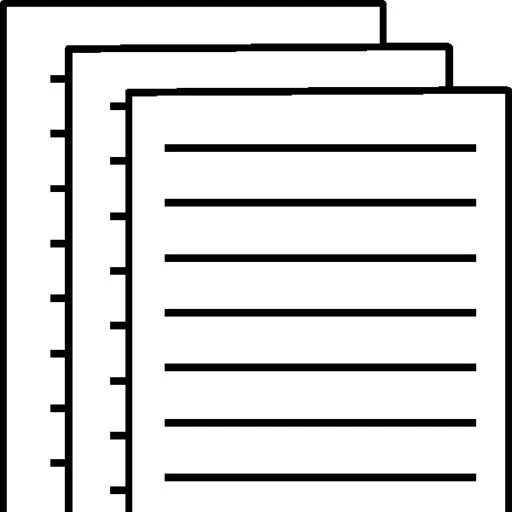## Estimation Techniques

Estimation Techniques

There are various methods that may be used to determine activity estimates with respect to resources, costs, and durations. Choose at least three estimation techniques that may be used for determining activity estimates for an IT project. Describe the pros and cons of each. Determine the most efficient estimation technique for an IT project; include an example or scenario to support your response.

However, the three estimation techniques that may be used for determining activity for an IT project will be Analogous Estimating, Parametric Estimating, and Three Point Estimates. To continue, these three can be perfect for the estimation of techniques to have methods to showcase the ability on how the techniques will work for these three examples thats given. In addition, the pros and cons for each examples are:

Pros for Analogous Estimating

you can estimate the duration of a project very quickly

accuracy of analogous estimation can be  depend on the degree of similarities between your project and the project you are comparing it with.

Cons for Analogous

this method is that the estimation may not always be accurate.

It can thus be assumed that the analogous estimation is 1 month.

Pros for Parametric Estimating

Parametric estimation is done on a per unit basis and uses the relation between variables to arrive at the cost or the duration.

parametric estimation stands to be more accurate.

Cons for Parametric Estimating

the measurement must be scalable in order to achieve the accuracy.

Pros for Three Point Estimates

Three-point estimating helps project managers make better estimates

managers using three-point estimating gain more granular control of how the end value is calculated.

Cons for Three Point Estimates

that it needs detail information and getting that information

it also takes time to do this estimate on point estimate

And the most efficient estimation technique will be Three point Estimate is because its the only one that can officially take charge on the other estimates.

Describe the accuracy level and the risk level of effort and the activities associated with each estimation technique you chose in Part 1 of this discussion. Determine the technique that would be efficient for the following:

The accuracy level  and risk level to my opinion will be in this way to my knowledge will be that the Level of Accuracy and the risk level attainable is closely related to the tools, techniques and processes used to capture and represent the objects being documented In the case of laser scanning, each scanner has a stated collection accuracy along by having the risk level and accuracy of it.

Systems development project= the techniques that will work for this one will be Analogous Estimating

High-risk software project = the techniques that will work for this one will be Three Point Estimates

Estimating a source code based project= the techniques that will work for this one will be Parametric Estimating.

To view and download a complete answer, scroll down to the bottom to pay